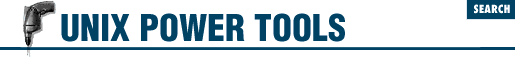#Chapter 45Shell Programming for the Initiated## 45.28 Quick Reference: expr

expr expr is a very handy tool in shell programming, since it provides the ability to evaluate a wide range of arithmetic, logical, and relational expressions. It evaluates its arguments as expressions and prints the result. expr is a standard UNIX utility; the GNU version is on the CD-ROM.

Here's the syntax. The [brackets] mean "optional"; don't type the brackets:

`expr` `arg1 operator arg2 ``[` `operator arg3 ``... ]`

Arguments and operators must be separated by spaces. In many cases, an argument is an integer, typed literally or represented by a shell variable. There are three types of operators: arithmetic, relational, and logical.

Exit status (44.7) values for expr are 0 if the expression evaluates non-zero and non-null, 1 if the expression evaluates to 0 or null, or 2 if the expression is invalid.

Arithmetic operators

Use these to produce mathematical expressions whose results are printed.

`+`

Add `arg2` to `arg1`.

`-`

Subtract `arg2` from `arg1`.

`*`

Multiply the arguments.

`/`

Divide `arg1` by `arg2`.

`%`

Take the remainder when `arg1` is divided by `arg2`.

Addition and subtraction are evaluated last, unless they are grouped inside parentheses. The symbols `*`, `(`, and `)` have meaning to the shell, so they must be escaped (preceded by a backslash or enclosed in quotes).

Relational operators

Use these to compare two arguments. Arguments can also be words, in which case comparisons assume a`<`z and A`<`Z. If the comparison statement is true, expr writes 1 to standard output (13.1); if false, it writes 0. The symbols `>` and `<` must be escaped.

`=`

Are the arguments equal?

`!=`

Are the arguments different?

`>`

Is `arg1` greater than `arg2`?

`>=`

Is `arg1` greater than or equal to `arg2`?

`<`

Is `arg1` less than `arg2`?

`<=`

Is `arg1` less than or equal to `arg2`?

Logical operators

Use these to compare two arguments. Depending on the values, the result written to standard output can be `arg1` (or some portion of it), `arg2`, or 0. The symbols `|` and `&` must be escaped.

`|`

Logical OR; if `arg1` has a non-zero (and non-null) value, the output is `arg1`; otherwise, the output is `arg2`.

`&`

Logical AND; if both `arg1` and `arg2` have a non-zero (and non-null) value, the output is `arg1`; otherwise, the output is 0.

`:`

Sort of like grep (27.1); `arg2` is a pattern to search for in `arg1`. `arg2` must be a regular expression in this case. If the `arg2` pattern is enclosed in `\( \)`, the output is the portion of `arg1` that matches; otherwise, the output is simply the number of characters that match. A pattern match always applies to the beginning of the argument (the `^` symbol is assumed by default).

### 45.28.1 Examples

Division happens first; output is 10:

`\$ `expr 5 + 10 / 2``

Addition happens first; output is 7 (truncated from 7.5):

`\$ `expr \( 5 + 10 \) / 2``

Add 1 to variable i; this is how variables are incremented in Bourne shell scripts:

``i=`expr "\$i" + 1```

Output 1 (true) if variable a is the string "hello":

`\$ `expr "\$a" = hello``

Output 1 (true) if variable b plus 5 equals 10 or more:

`\$ `expr "\$b" + 5 \>= 10``

In the examples below, variable p is the string "version.100". This command returns the number of characters in p:

`\$ `expr "\$p" : '.*'`   Output is 11`

Match all characters and print them:

`\$ `expr "\$p" : '\(.*\)'`   Output is "version.100"`

Output the number of lowercase letters matched:

`\$ `expr "\$p" : '[a-z]*'`   Output is 7`

Match a string of lowercase letters:

`\$ `expr "\$p" : '\([a-z]*\)'`   Output is \"version"`

Truncate `\$x` if it contains five or more characters; if not, just output `\$x`. (Logical OR uses the second argument when the first one is 0 or null; i.e., when the match fails.)

`\$ `expr "\$x" : '\(.....\)'  "\$x"``

- DG from O'Reilly & Associates' UNIX in a Nutshell (SVR4/Solaris)45.27 Turn Off echo for "Secret" Answers45.29 Testing Characters in a String with expr